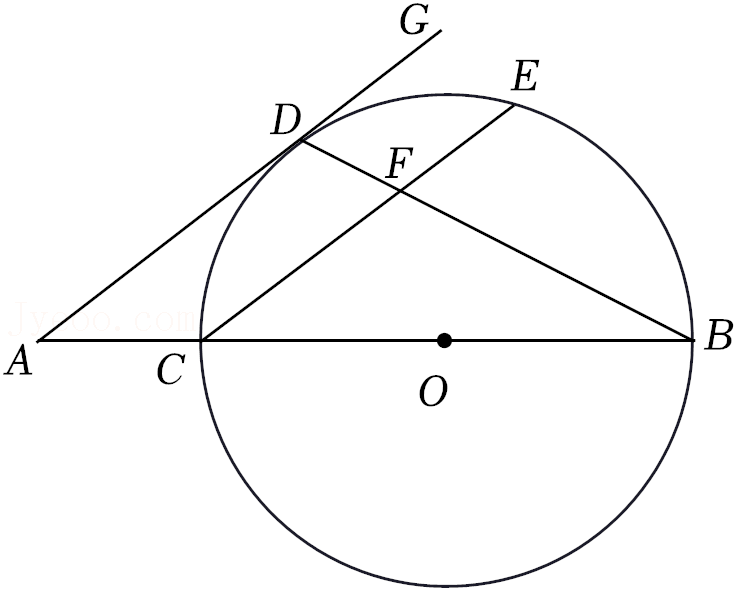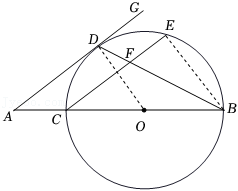(1) 求证: $\mathrm{AD}$ 是 $\odot O$ 的切线;
(2) 若 $\mathrm{EF}=3, \mathrm{CF}=5, \tan \angle \mathrm{GDB}=2$, 求 $\mathrm{AC}$ 的长.【答案】 26.(1)证明：如图，连接$OD$，$BE$$\because$ 点 $\mathrm{D}$ 为 $\widehat{C E}$ 的中点,
$$\therefore \widehat{C D}=\widehat{\mathrm{ED}} \text {, }$$
$$\therefore \angle \mathrm{CBD}=\angle \mathrm{EBD} \text {, }$$
$$\because \mathrm{OB}=\mathrm{OD} \text {, }$$
$$\therefore \angle \mathrm{ODB}=\angle \mathrm{CBD} \text {, }$$
$$\therefore \angle \mathrm{ODB}=\angle \mathrm{EBD} \text {, }$$
$$\therefore \mathrm{OD} / / \mathrm{BE} \text {, }$$
$\because B C$ 为 $\odot O$ 的直径,
$$\therefore \angle \mathrm{CEB}=90^{\circ} \text {, }$$

\begin{aligned} & \therefore \mathrm{CE} \perp \mathrm{BE}, \\ & \therefore \mathrm{OD} \perp \mathrm{CE}, \\ & \because \mathrm{AD} / / \mathrm{CE}, \\ & \therefore \mathrm{AD} \perp \mathrm{OD}, \\ & \because \mathrm{OD} \text { 是 } \odot \mathrm{O} \text { 的半径, } \\ & \therefore \mathrm{AD} \text { 是 } \odot \mathrm{O} \text { 的切线; } \end{aligned}

(2) 解: $\because \mathrm{DG} / / \mathrm{CE}$,
\begin{aligned} & \therefore \angle \mathrm{BFE}=\angle \mathrm{GDB}, \angle \mathrm{A}=\angle \mathrm{ECB}, \\ & \because \tan \angle \mathrm{GDB}=2, \\ & \therefore \tan \angle \mathrm{BFE}=2, \\ & \text { 在 } \mathrm{Rt} \triangle \mathrm{BEF} \text { 中, } \mathrm{EF}=3, \tan \angle \mathrm{BFE}=\frac{\mathrm{BE}}{\mathrm{EF}}, \\ & \therefore \mathrm{BE}=6, \\ & \because \mathrm{EF}=3, \mathrm{CF}=5, \\ & \therefore \mathrm{CE}=\mathrm{EF}+\mathrm{CF}=8, \\ & \therefore \mathrm{BC}=\sqrt{\mathrm{CE}}{ }^2+\mathrm{BE}^2=10, \\ & \therefore \mathrm{OD}=\mathrm{OC}=5, \\ & \text { 在 } \mathrm{Rt} \triangle \mathrm{BCE} \text { 中, } \sin \angle \mathrm{ECB}=\frac{\mathrm{BE}}{\mathrm{BC}}=\frac{6}{10}=\frac{3}{5}, \\ & \therefore \sin \mathrm{A}=\sin \angle \mathrm{ECB}=\frac{3}{5}, \\ & \text { 在 } \mathrm{Rt} \triangle \mathrm{AOD} \text { 中, } \sin \mathrm{A}=\frac{\mathrm{OD}}{\mathrm{OA}}=\frac{3}{5}, \mathrm{OD}=5, \\ & \therefore \mathrm{OA}=\frac{25}{3}, \\ & \therefore \mathrm{AC}=\mathrm{OA}-\mathrm{OC}=\frac{10}{3} . \end{aligned}